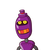# express the equation 3x=7y-21 in the form of ax+by+c=0 and wrote the values of a,b, and c​

express the equation 3x=7y-21 in the form of ax+by+c=0 and wrote the values of a,b, and c​

### 2 thoughts on “express the equation 3x=7y-21 in the form of ax+by+c=0 and wrote the values of a,b, and c​”

1.## and c=21

Step-by-step explanation:

3x=7y-21

Subtracting 7y from both sides

3x-7y=7y-7y-21

3x-7y=-21

3x-7y+21=-21+21

### 3x-7y+21=0

Comparing with

ax+by+c=0

a=3

b=-7

and c=21

2.